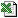PolyReg (Degree, YValues, XValues, [MinRSqrdOpt], [MaxDegPoly])
Polynomial Regression. Fit a polynomial to a set of data. Will detect the best order of polynomial if required (if Degree=0).
Fits a set of Coefficients using Least Squares to a polynomial of degree Degree, to a maximum of 20 (a+bx^0 ... ux^20).
###### Key Points

We advise bit of care when fitting a polynomial. The algorithm we use is not the state of the art in polynomial fitting. Mathematicians will note that an algorithm using orthogonal reduction or singular value decomposition would perhaps be more robust than our Gaussian solver. Nonetheless, our experience with this function is that the issue is really with the problem you are asking the algorithm to solve. It generally WILL provide a polynomial that fits pretty well your input data, but the algorithm is not responsible for what happens to the curve inbetween your data points! Sometimes, for example, the 2nd and 3rd coefficients might be very large but of opposite sign, meaning that the algorithm has successfully fitted the data but may have come up with a wildly oscillating equation. The answer to this problem is to specify more input data, so as to define the curve a bit more in order that the function is less likely to get led astray.

###### Key Points

Our recommendations would be:

• Use the lowest order of degree.
• Have good look at the output equation, perhaps chart it, to test for "sensibleness".
• It"s a good idea to set a value of MinRSqrdOpt (e.g. 90%), so you can deliberately force the function to throw an error if it can"t find a good enough fit.

###### Formula Derivation

The algorithm uses the Least Squares method. To solve the matrix for the coefficients a Gauss algorithm is used.

###### Application

This function is always used as an array function, because you need an array of parameters returned. In fact, you need as many parameters as you have coefficients. The coefficients are the "powers" of the polynomial. For example, for a second order polynomial y=a+bx+cx^2, the coefficients are a,b and c. The maximum degree of polynomial allowed is 20 (a+bx...ux^20).

###### Tip

Set MinRSqrdOpt to something like 0.9 (90%) to force the function to throw an error if it can"t find a good enough fit. You don"t really want it to give you an equation if the goodness if fit is simply appalling!

###### Tip

Setting a Degree of zero means that the function will return the best fit it can using a Degree that can vary between 1 and 20.

###### ExamplePolyReg.xls
###### Templatescurve_poly.xls